# GMAT Avengers Study Group: Quadrilaterals:

by on October 3rd, 2014

A polygon is a closed 2D shape with all the straight lines. A quadrilateral is a polygon with 4 sides or edges and four vertices or corners and the sum of its angles equals 360°.

There are 5 types of quadrilaterals and one should be familiar with each of them: Parallelogram, Rectangle, Square, Rhombus, and Trapezoid

Let’s discuss a few properties of each as we learn them.

## Parallelogram

It has opposite sides parallel. It has two pairs of parallel sides. The opposite angles are equal and the opposite sides have equal length.Properties

• Opposite sides of a parallelogram are equal in length.
• Opposite angles of a parallelogram are equal in measure.
• Opposite sides of a parallelogram will never intersect.
• The diagonals of a parallelogram bisect each other.
• Consecutive angles are supplementary, add to 180°.
• The area, A, of a parallelogram is, where b is the base of the parallelogram and h is its height.
• The area of a parallelogram is twice the area of a triangle created by one of its diagonals.

• A rectangle is a parallelogram but with all angles fixed at 90°.
• A rhombus is a parallelogram but with all sides equal in length.
• A square is a parallelogram but with all sides equal in length and all angles fixed at 90°.

## Rectangle

It is a special type of quadrilateral that has opposite sides parallel and equal. The opposite angles are equal and each equal 90°.Properties

• Opposite sides are parallel and congruent.
• The diagonals bisect each other and are of same length.
• A square is a special case of a rectangle where all four sides are the same length.
• It is also a special case of a parallelogram but with extra limitation that the angles are fixed at 90°.
• Each diagonal bisects the other. In other words, the point where the diagonals intersect (cross), divides each diagonal into two equal parts.
• Each diagonal divides the rectangle into two congruent right triangles. Because the triangles are congruent, they have the same area, and each triangle has half the area of the rectangle.
• The formula for calculating the diagonal is given bywhere: w is the width of the rectangle, h is the height of the rectangle.
• The area of a rectangle is given by the formula.

Other Interesting Facts

• A square is a special case of a rectangle where all four sides are the same length. Adjust the rectangle above to create a square.
• It is also a special case of a parallelogram but with extra limitation that the angles are fixed at 90°.

## Square

It is a special type of quadrilateral with all sides equal and all internal angles 90°.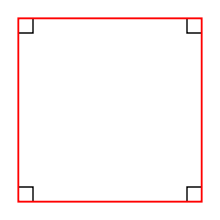Properties

• If the diagonals of a rhombus are equal, then that rhombus must be a square. The diagonals of a square are(about 1.414) times the length of a side of the square.
• A square can also be defined as a rectangle with all sides equal, or a rhombus with all angles equal, or a parallelogram with equal diagonals that bisect the angles.
• If a figure is both a rectangle (right angles) and a Rhombus (equal edge lengths), then it is a Square. (Rectangle (four equal angles) + Rhombus (four equal sides) = Square)
• If a circle is circumscribed around a square, the area of the circle is π/2 (about 1.57) times the area of the square.
• If a circle is inscribed in the square, the area of the circle is π/4 (about 0.79) times the area of the square.
• A square has a larger area than any other quadrilateral with the same perimeter.
• Like most quadrilaterals, the area is the length of one side times the perpendicular height. So in a square this is simply:, where S is the length of one side.
• The “diagonals” method. If you know the lengths of the diagonals, the area is half the product of the diagonals. Since both diagonals are congruent (same length), this simplifies to:, where d is the length of either diagonal.
• Each diagonal of a square is the perpendicular bisector of the other. That is, each cuts the other into two equal parts, and they cross and right angles (90°).
• The length of each diagonal iswhere S is the length of any one side.

A square is both a rhombus (equal sides) and a rectangle (equal angles) and therefore has all the properties of both these shapes, namely:

• The diagonals of a square bisect each other.
• The diagonals of a square bisect its angles.
• The diagonals of a square are perpendicular.
• Opposite sides of a square are both parallel and equal.
• All four angles of a square are equal. (Each is 360/4 = 90°, so every angle of a square is a right angle.)
• The diagonals of a square are equal.

Other Interesting Facts

• a rectangle but with adjacent sides equal
• a parallelogram but with adjacent sides equal and the angles all 90°
• a rhombus but with angles all 90°

## Rhombus

It is a quadrilateral with all four sides equal in lengthProperties

• A rhombus is actually just a special type of parallelogram. Recall that in a parallelogram each pair of opposite sides are equal in length. With a rhombus, all four sides are the same length. It therefore has all the properties of a parallelogram.
• The diagonals of a rhombus always bisect each other at 90°.
• There are several ways to find the area of a rhombus. The most common is:. The other one is the “diagonals” method. Another simple formula for the area of a rhombus when you know the lengths of the diagonals. The area is half the product of the diagonals. As a formula:, where d1 is the length of a diagonal d2 is the length of the other diagonal.

## Trapezoid

A quadrilateral which has at least one pair of parallel sides.Properties

• Base - One of the parallel sides. Every trapezoid has two bases.
• Leg - The non-parallel sides are legs. Every trapezoid has two legs.
• Altitude - The altitude of a trapezoid is the perpendicular distance from one base to the other. (One base may need to be extended).
• If the legs are parallel, it now has two pairs of parallel sides, and is a parallelogram.
• Median - The median of a trapezoid is a line joining the midpoints of the two legs.
• The median line is always parallel to the bases.
• The length of the median is the average length of the bases, or using the formula:• The median line is halfway between the bases.
• The median divides the trapezoid into two smaller trapezoids each with half the altitude of the original.
• Area - The usual way to calculate the area is the average base length times altitude. The area of a trapezoid is given by the formulawhere a, b are the lengths of the two bases h is the altitude of the trapezoid.
• The area of a trapezoid is.

A quick recap of the articles that we shared on our Facebook Event Page before we share the practice problems

## Problem Solving Practice

1) The length of a certain rectangle is 4 inches longer than its width. If the area of the rectangle is 221 square inches, then the length of the rectangle’s diagonal, in inches, is?

(A) between 19 and 20

(B) between 20 and 21

(C) between 21 and 22

(D) between 22 and 23

(E) between 23 and 24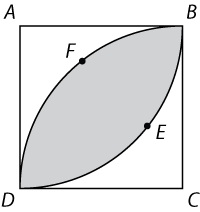2) In the figure shown, ABCD is a square with side length. Two quarter-circles BED and DFB are centered at A and C, respectively. What is the area of the shaded region?

(A) 50π-25

(B) 25π−50

(C) 50π/3

(D) 25π/3

(E) 25π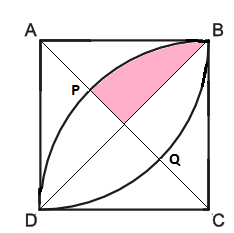3) As shown in figure, square ABCD has arc BPD centered at C and arc BQD centered at A. If AB = 4, the area of the shaded region is?

(A)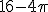(B)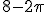(C)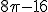(D)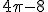(E)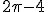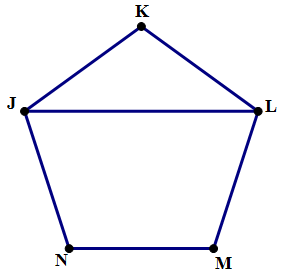4) In the figure above, JKLMN is a regular pentagon. Find the measure of ∠JLK.

(A) 24°

(B) 36°

(C) 48°

(D) 60°

(E) 72°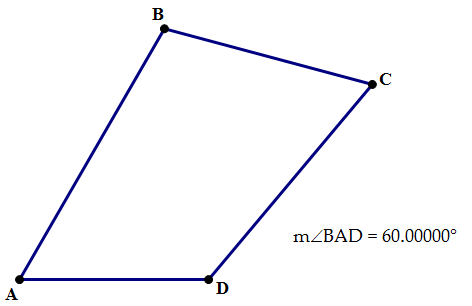5) In the figure above, in irregular quadrilateral ABCD, ∠A = 60°, ∠D is twice ∠C, and ∠B is 40° more than ∠C.  Find the measure of ∠B

(A) 65°

(B) 85°

(C) 105°

(D) 120°

(E) 135°

## Data Sufficiency Practice

1) Sides AB, BC, and CD of quadrilateral ABCD all have length 10. What is the area of quadrilateral ABCD?

(A) BC is parallel to AD.

(B) Diagonal AC, which lies inside the quadrilateral, has length 10√3.

2) The perimeter of square ABCD is half of the perimeter of quadrilateral EFGH.  What is the perimeter of quadrilateral EFGH?

(A) a line segment drawn from the center of ABCD to the midpoint of BC is 2 inches long

(B) a line segment drawn from the center of EFGH to the midpoint of FG is 4 inches long3) In the trapezoid above, with sides abc, and 1, what is the value of ac?

(A)(B)4) What is the perimeter of rectangle R?

(A) R is a square.

(B) The area of R is 36.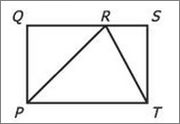5) In the figure shown, what is the area of triangular region PRT?

(A) The area of rectangular region PQST is 24

(B) The length of line segment RT is 5

6) What is the area of the quadrilateral with vertices A, B, C, and D?

(A) The perimeter of ABCD is equal to 16.

(B) Quadrilateral ABCD is a rhombus.

7) What is the area of square ABCD ?

(A) The length of the side of square ABCD is 2.

(B) For square EFGH, which has sides that are 6 longer than those of square ABCD, the ratio of the perimeter to the area is the reciprocal of the corresponding ratio for square ABCD

8) Is quadrilateral ABCD a rhombus?

(A) Line segments AC and BD are perpendicular bisectors of each other.

(B) AB = BC = CD = AD

Problem Solving

1. C
2. B
3. E
4. B
5. C

Data Sufficiency

1. E - Statements (1) and (2) TOGETHER are NOT sufficient to answer the question asked, and additional data are needed.
2. A – Statement (1) ALONE is sufficient, but statement (2) alone is not sufficient.
3. A – Statement (1) ALONE is sufficient, but statement (2) alone is not sufficient.
4. C – BOTH statements TOGETHER are sufficient, but NEITHER statement ALONE is sufficient.
5. A – Statement (1) ALONE is sufficient, but statement (2) alone is not sufficient.
6. E – Statements (1) and (2) TOGETHER are NOT sufficient to answer the question asked, and additional data are needed
7. D – EACH statement ALONE is sufficient
8. D - EACH statement ALONE is sufficient

Make sure you check out the event Facebook page to see all the comments that were being exchanged during the live session.

***

EXCITING NEWS!! “The GMAT Avengers Guide” is now available! This eBook is a collection of transcripts, tips, strategies, recommended readings, best practices, and challenge problems to help you beat the GMAT and achieve your dream score. Download your FREE copy NOW!

RSVP NOW if you want to join me and fellow GMAT Avengers take on CR assumption questions.

•Hi rahul ,
I am not on facebook , so how to join the events because i am unable to join them.

•Hey Ankita - Hope you are doing good. Our Study Group sessions happen every week on Facebook only. So, one needs to have an account created on it in order to join us for the sessions. That way, you will be able to see all the comments, discussions that happened throughout the session.

Could you please create one ? Else, you will have the Transcript (as the one you see above) where we post all the relevant theory, questions that we shared throughout the session. Does that help ?

Please let our Team or I know in case you need anything else.

•Hey Rahul,

In case of problem solving Q)4 , assume angle KJL=x, So angle KLJ should also be x ( KJL is an isosceles triangle since sides KJ= KL as it is a regular pentagon ) . Angle JKL = 72 ( 360/5). Hence, x+x+72= 180 giving x= 54 degrees ( also angle JLK ) . Please clarify.